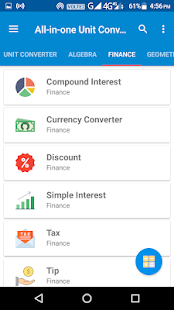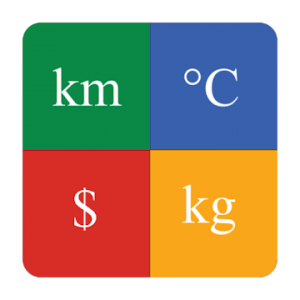All-in-one Calculator and Unit Converter is an easy to use app with features that you need.

This app included 15+ Unit Converters, Ideal Body Weight Calculator, Tip Calculator and many other features that you will love.

List of features that are supported:
1) General Calculator – Supports general mathematical operations such as addition, subtraction, multiplication, division, square root, and power
2) Algebra Calculator – Supports Equation Solver, Fractions, GCF / LCM, Percentage
3) Unit Converter – Supports conversion of units of Angle, Area, Data Storage, Data Transfer, Energy, Length, Number System, Pressure, Shoe Size, Speed, Temperature, Time, Volume, Weight
4) Health Calculators – Supports Basal Metabolic Rate (BMR), Body Mass Index (BMI), Body Fat Percentage, Calorie Intake, Ideal Body Weight
5) Finance Calculators – Supports Compound Interest, Discount, Simple Interest, Tip Calculator
6) Geometry Calculators – Supports Perimeter, Area, Volume, Surface Area Calculators along with Heron’s Formula Calculator
7) Time Calculators – Age Calculator, Elapsed Time Calculator (for both dates and times)

What’s New:
* Icon Fix
* Minor UI Improvements

Screenshots

•••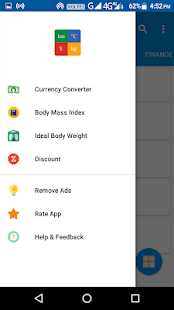•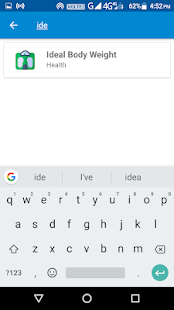•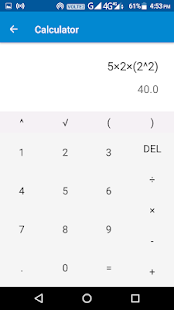•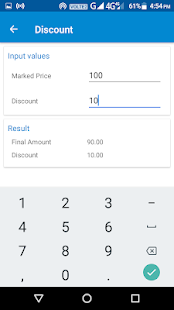•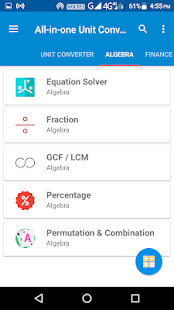•